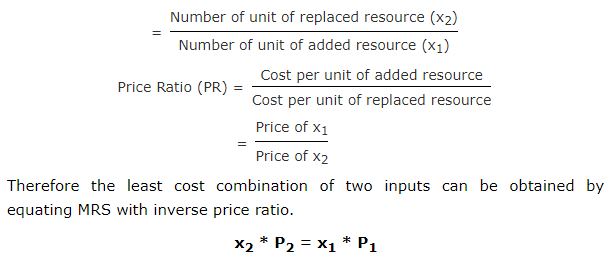# Isoquants, Optimal Combination of Inputs

Isoquants are a geometric representation of the production function. The same level of output can be produced by various combinations of factor inputs. The locus of all possible combinations is called the ‘Isoquant’.

Characteristics of Isoquant

• An isoquant slopes downward to the right.
• An isoquant is convex to origin.
• An isoquant is smooth and continuous.
• Two isoquants do not intersect.

Types of Isoquants

The production isoquant may assume various shapes depending on the degree of substitutability of factors.

Linear Isoquant

This type assumes perfect substitutability of factors of production. A given commodity may be produced by using only capital or only labor or by an infinite combination of K and L.

Input-Output Isoquant

This assumes strict complementarily, that is zero substitutability of the factors of production. There is only one method of production for any one commodity. The isoquant takes the shape of a right angle. This type of isoquant is called “Leontief Isoquant”.

Kinked Isoquant

This assumes limited substitutability of K and L. Generally, there are few processes for producing any one commodity. Substitutability of factors is possible only at the kinks. It is also called “activity analysis-isoquant” or “linear-programming isoquant” because it is basically used in linear programming.

Least Cost Combination of Inputs

A given level of output can be produced using many different combinations of two variable inputs. In choosing between the two resources, the saving in the resource replaced must be greater than the cost of resource added. The principle of least cost combination states that if two input factors are considered for a given output then the least cost combination will have inverse price ratio which is equal to their marginal rate of substitution.

Marginal Rate of Substitution

MRS is defined as the units of one input factor that can be substituted for a single unit of the other input factor. So MRS of x2 for one unit of x1 is −## 2 thoughts on “Isoquants, Optimal Combination of Inputs”

error: Content is protected !!